ConceptDraw
DIAGRAM 16

# Multi Layer Venn Diagram. Venn Diagram Example

A set is one of the key concepts of mathematics. The concept of the set is usually taken as one of the axiomatic concepts, that is, it can not be reduced to other concepts, and therefore has no definition. However, you can give a description of the set, as a collection of different elements, perceived as a whole.

Venn diagram shows all the possible relationships between sets or events of a certain family.

If element X belongs to a set M that is denoted by X ∈ M, and non-membership - X ∉ M. If the sets A and B are composed of the same elements, it is said that they are equal: A = B. Otherwise, the A and B sets are not equal (is denoted by A ≠ B). The set A is called a subset of the set B (denoted A ⊆ B), if every element of the set A is contained in the set B. In this case we also may say that B comprises the A or is a superset of A (denoted by B ⊇ A). The set A is called a proper subset of B (denoted A ⊂ B), if A ⊆ B and A ≠ B, i.e. B has elements that are not contained in A.

A set containing a finite number of elements is called finite, otherwise - infinite. The number of elements of a finite set M is called its power.

The set which doesn’t contain any elements is called empty (denoted by ∅). By definition, ∅ is equal to zero. It is believed that the empty set is a subset of any set. The basic operations on sets are union, intersection, difference and addition.

Union of sets A and B (denoted by AUB) is a set of elements, each of which belongs to either A or B. Thus, a random element X ϵ АUВ if and only if either X ϵ A, and X ϵ B.

Intersection of sets A and B (denoted by A∩B) is the set consisting of all those and only those elements which belong to both set A and set B.

Difference between the sets A and B (denoted by A B) is the set of all those and only those elements of A that are not contained in B.

A logical complement of A (denoted Ā or ¬A) is the set of all those elements that do not belong to the set A.

This sample was created in ConceptDraw DIAGRAM diagramming and vector drawing software using the Business Diagrams Solution from the Management area of ConceptDraw Solution Park.

This example shows the radially-symmetrical 5 set Venn Diagram. The Venn Diagrams visualize all possible logical relations between several sets and are widely used in mathematics, logic, statistics, marketing, sociology, philosophy, etc.Example 1. Multi Layer Venn Diagram

Using the ready-to-use predesigned objects, samples and templates from the Business Diagrams Solution for ConceptDraw DIAGRAM you can create your own professional-looking Venn Diagrams quick and easy.

The Venn Diagrams produced with ConceptDraw DIAGRAM are vector graphic documents and are available for reviewing, modifying, and converting to a variety of formats (image, HTML, PDF file, MS PowerPoint Presentation, Adobe Flash or MS Visio).

### ER Diagram Programs for Mac →

ConceptDraw DIAGRAM compatible Mac OS X software extended with powerful Entity-Relationship Diagram (ERD) Solution from the Software Development Area for ConceptDraw Solution Park is one of the famous ER Diagram Programs for Mac. It gives the perfect ability to draw ER diagrams for visually describing databases with Chen's and Crow's Foot notation icons.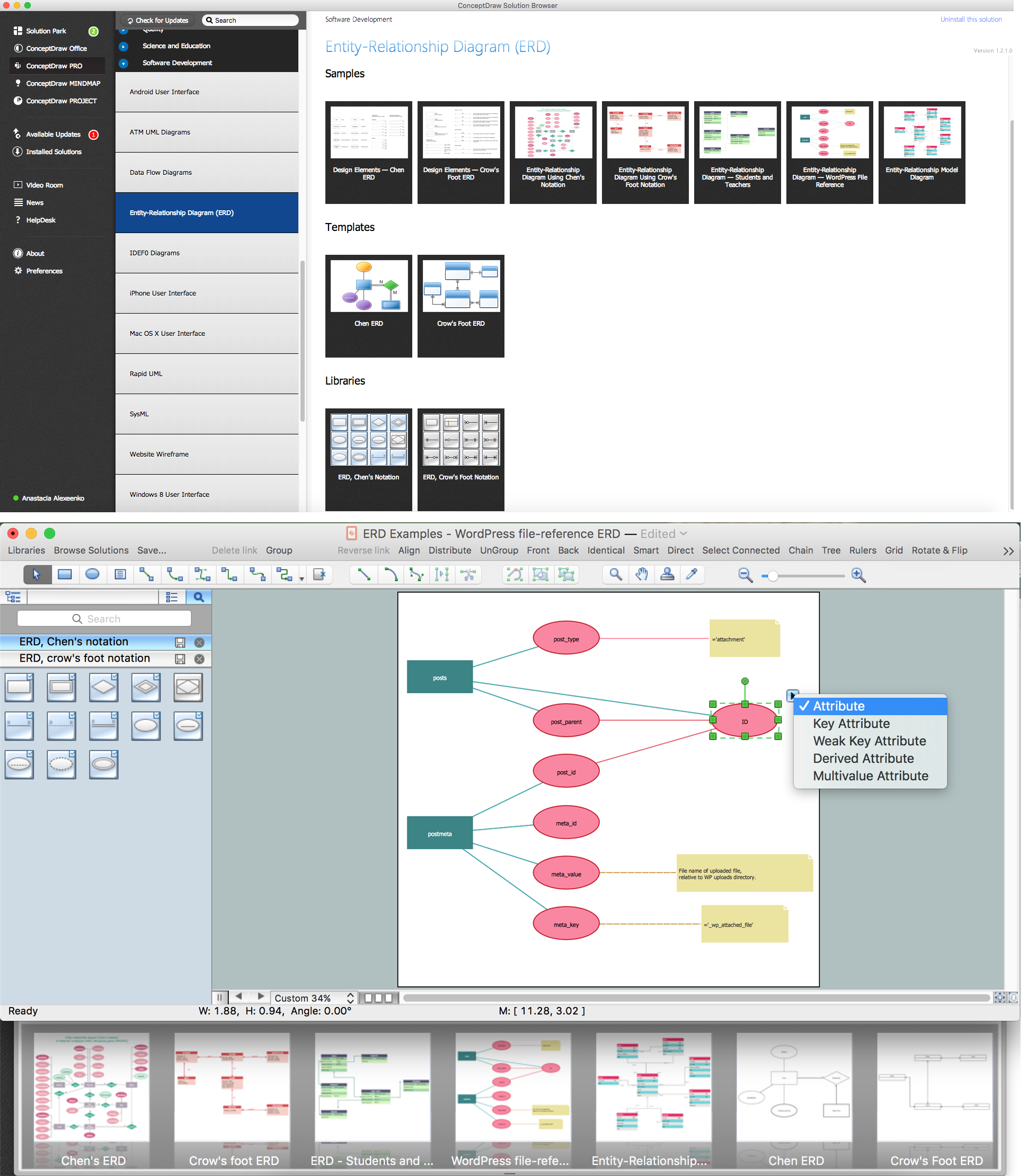Picture: ER Diagram Programs for Mac
Related Solution:

### Column Chart Software →

ConceptDraw Column Chart software allows drawing column charts using predesigned objects or drawing tools. In ConceptDraw Column Chart software you can find a complete set of column chart tools and objects.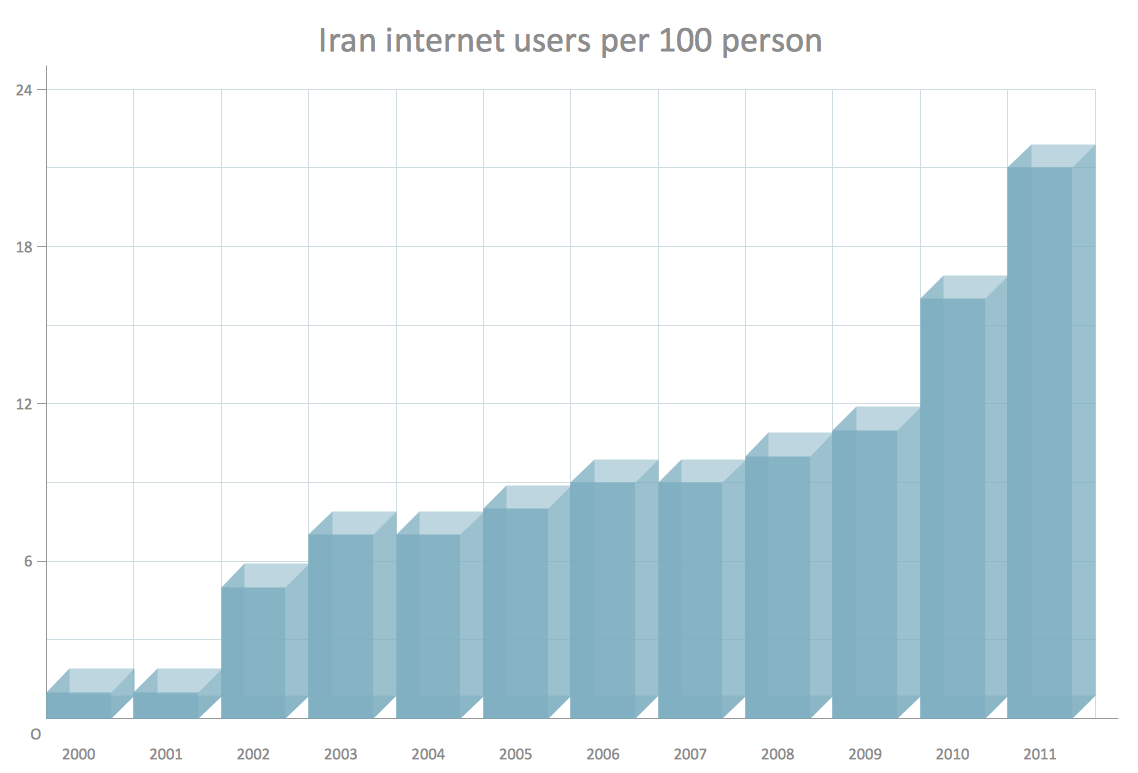Picture: Column Chart Software
Related Solution:

### Fire Evacuation Plan Template →

Have you ever created the fire plans on the base of Fire Evacuation Plan Template? It's incredibly convenient and time-saving way. Let's try to make sure this with Fire and Emergency Plans solution for ConceptDraw DIAGRAM software.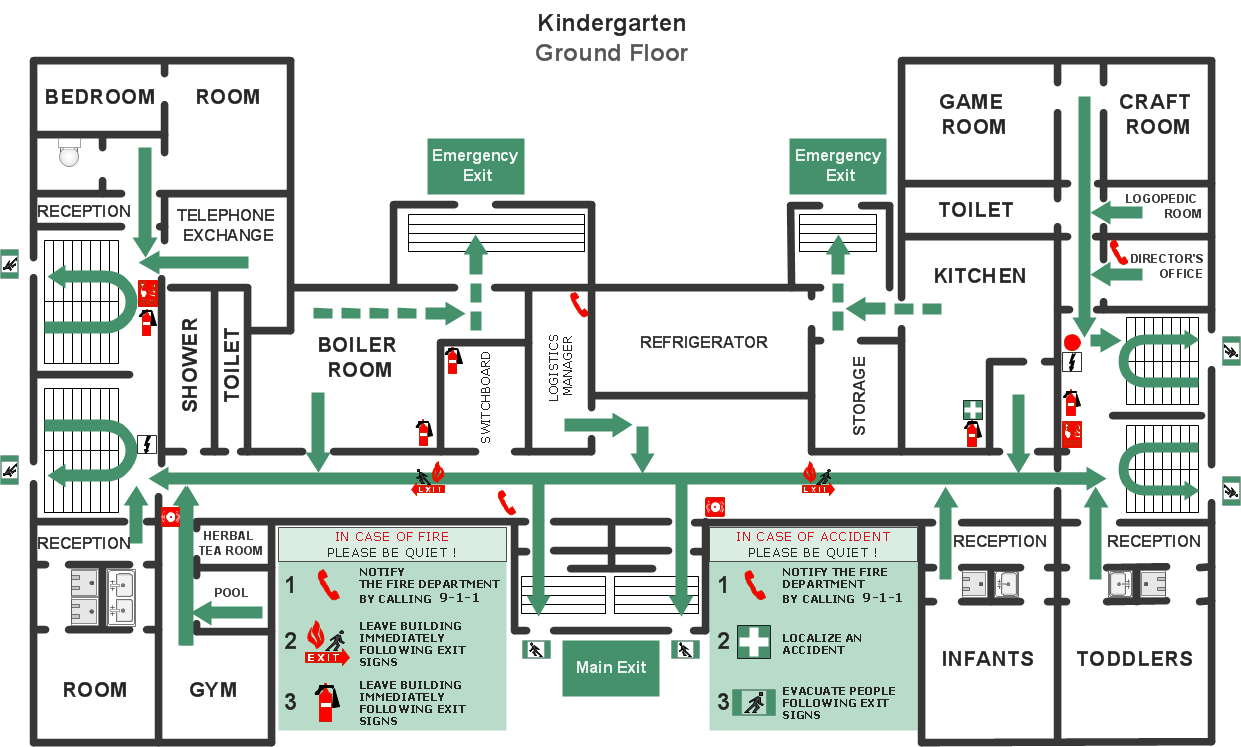Picture: Fire Evacuation Plan Template
Related Solution:

### Functional Flow Block Diagram →

You need to draw a Functional Flow Block Diagram? You are an artist? Now it doesn't matter. With Block Diagrams solution from the "Diagrams" area for ConceptDraw Solution Park you don't need more to be an artist to design the Functional Flow Block Diagram of any complexity.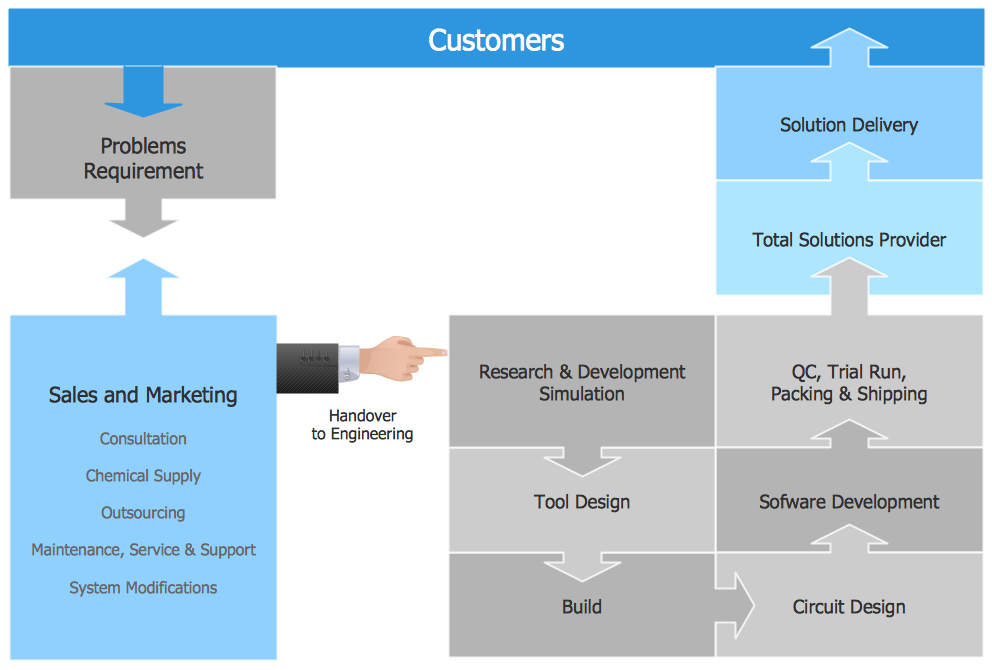Picture: Functional Flow Block Diagram
Related Solution:

### Context Diagram Template →

This template shows the Context Diagram. It was created in ConceptDraw DIAGRAM diagramming and vector drawing software using the Block Diagrams Solution from the “Diagrams” area of ConceptDraw Solution Park. The context diagram graphically identifies the system. external factors, and relations between them. It’s a high level view of the system. The context diagrams are widely used in software engineering and systems engineering for designing the systems that process the information.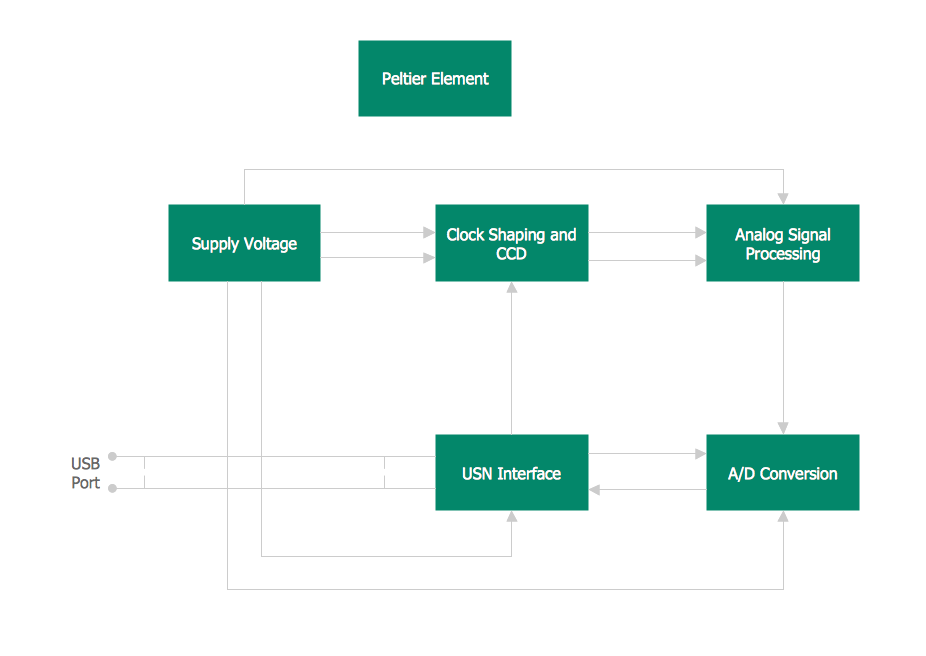Picture: Context Diagram Template
Related Solution:

### How to Simplify Flow Charting — Cross-functional Flowchart →

In ConceptDraw DIAGRAM it really is sp simple to draw even the most complex cross-functional flowcharts. Use the RapidDraw technology and Cross-Functional libraries in ConceptDraw DIAGRAM for the best results.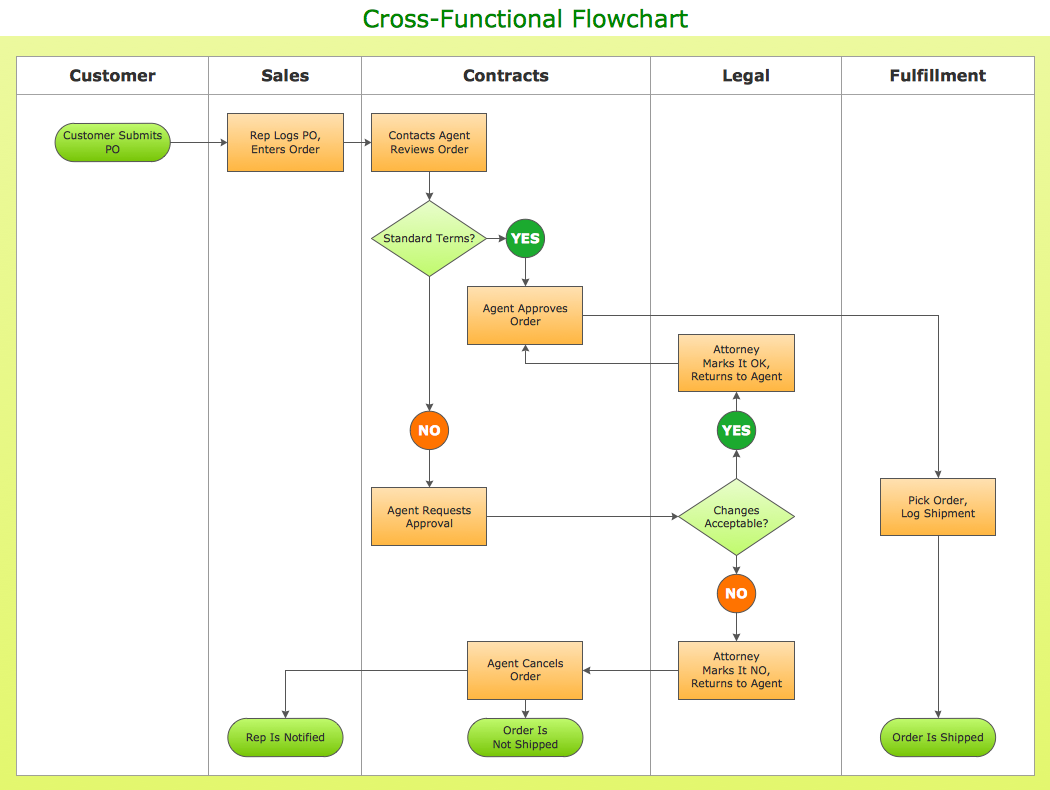Picture: How to Simplify Flow Charting — Cross-functional Flowchart
Related Solution:

### UML Package Diagram. Design Elements →

UML Package Diagram illustrates the functionality of a software system. ConceptDraw has 393 vector stencils in the 13 libraries that helps you to start using software for designing your own UML Diagrams. You can use the appropriate stencils of UML notation from UML Package library.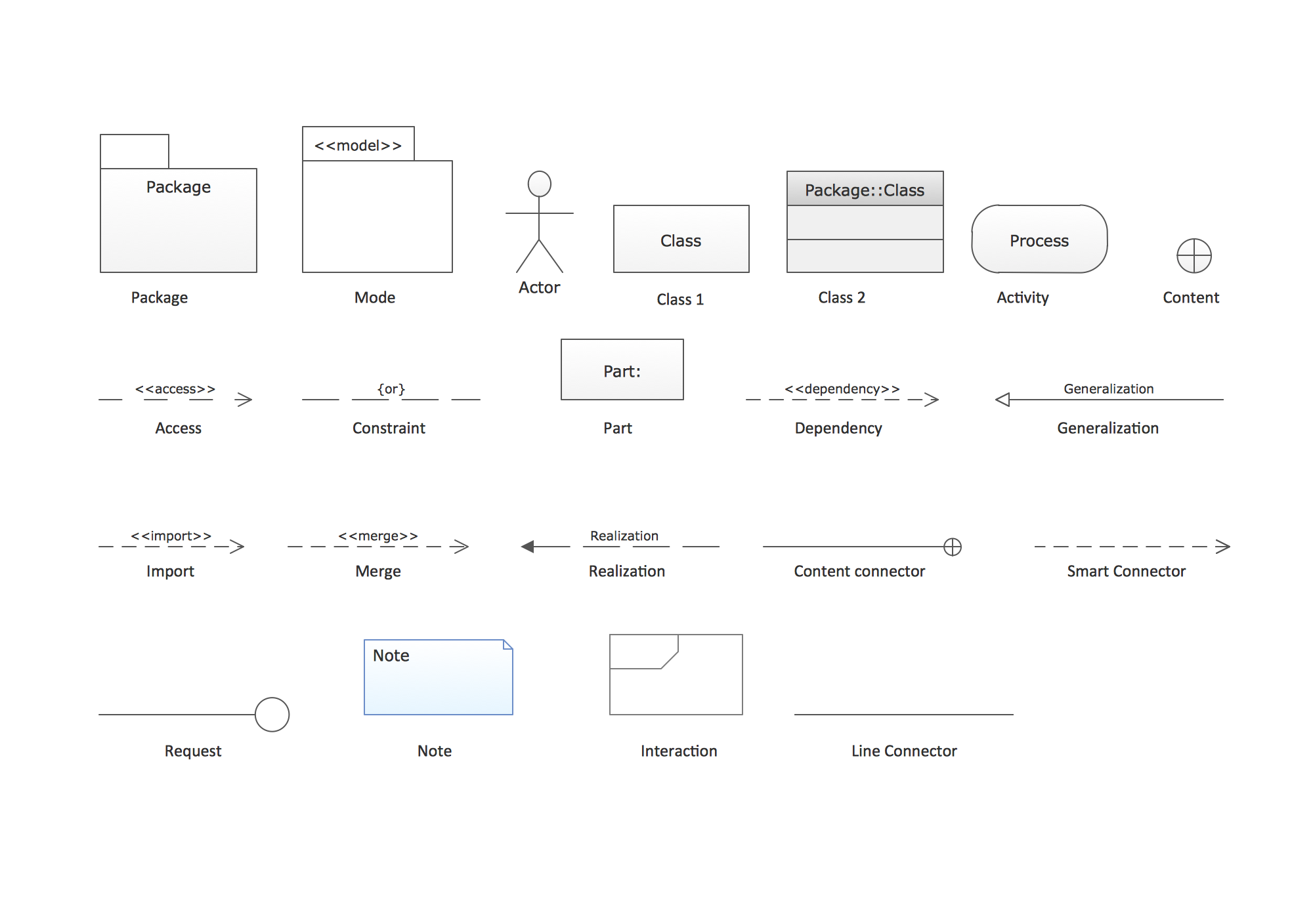Picture: UML Package Diagram. Design Elements
Related Solution:

### Pyramid Diagram →

A triangular graphic representing Graham's "hierarchy of disagreement", based on Paul Graham's March 2008 essay "How to Disagree".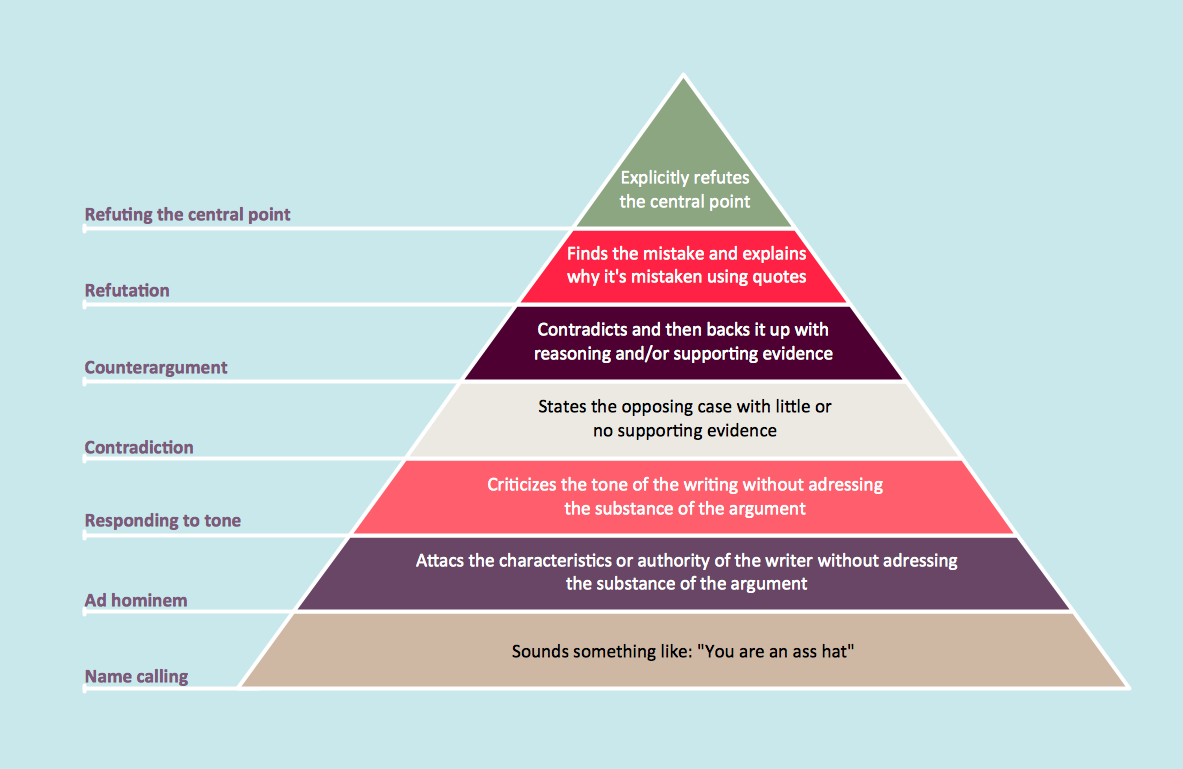Picture: Pyramid Diagram
Related Solutions:
ConceptDraw
DIAGRAM 16